## Superfluid Helium

1. Helium stays fluid down to T=0°K, since
• The van der Waals attraction between helium atoms is weak (closed electronic shells)
• Quantum fluctuations are large (light mass)
• Phase diagram of helium: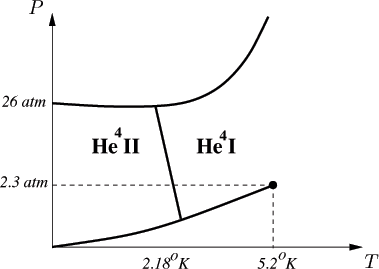• The isotope He4 (but not He3) undergoes a phase transition to a new state at T=2.18°K
• In evaporative cooling boiling stops, and He-II is a quiscent
•
2. Some unusual properties of He-II:
• The puzzle of its viscosity:
• It flows through the finest capillaries with no apparent resistance
• There is a finite drag of fluid in torsional experiments (Keesom, Andronikashvilli)
•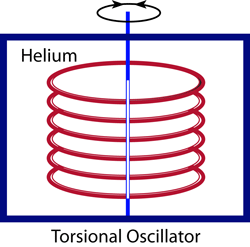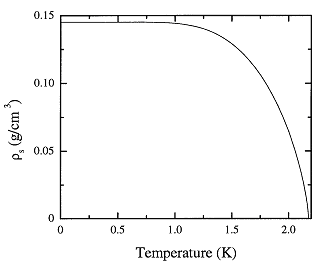• Thermo-mechanical couplings:
• No boiling bubbles
• Heating of a pressurized compartment in the superflow experiment
•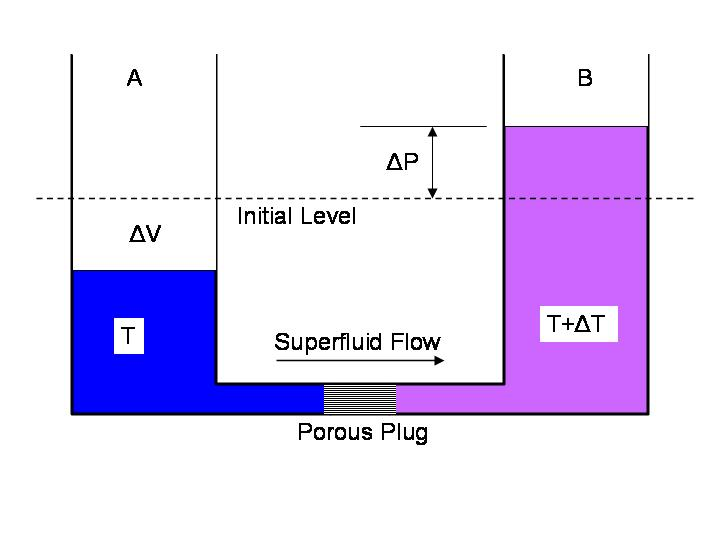• The fountain effect
•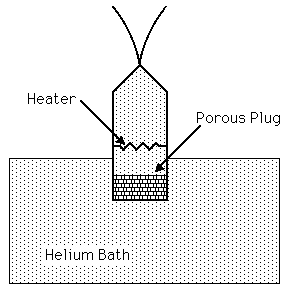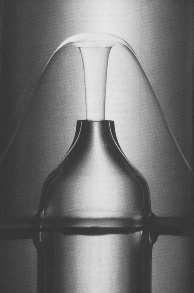3. Is superfluidity related to Bose-Einstein Condensation (BEC)? (Fritz London, 1938)
•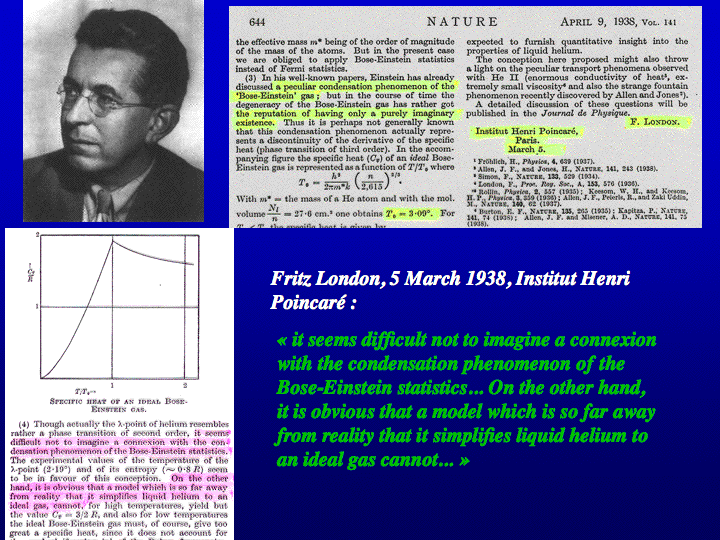• It does not occur in the fermionic isotope of He3
• The predicted BEC transition temperature of  Tc=3.13°K at the density of helium is not far off.
• Lazlo Tisza's two fluid model can explain the thermo-mechanical effects.
••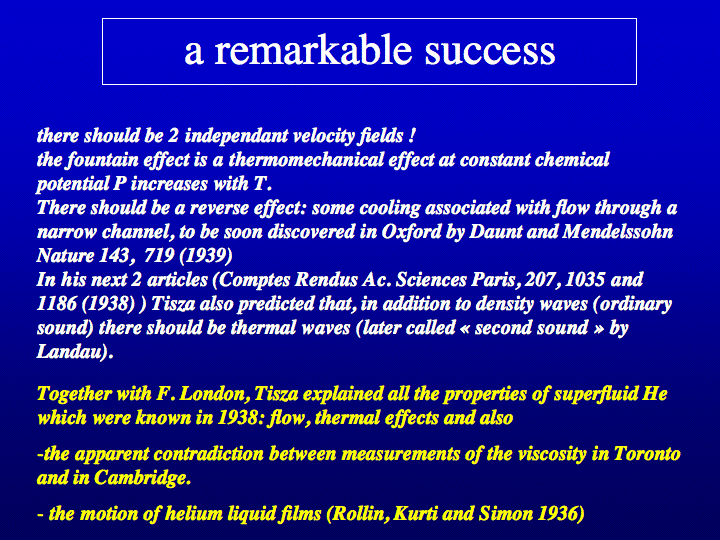4. Key differences of helium superfluidity and BEC:
• Interactions: He-II is a practically incompressible liquid due to hard-core interactions, while the ideal BEC has no compressibility!
• Differences in the heat-capacity curves:
• There is a λ-like logarithmic divergence in heat capacity, as opposed to the finite hump in BEC
• Heat capacity vanishes as T^{3} at low temperatures, as opposed to T^{3/2} for BEC
• Differences in the superfluid fraction:
• The superfluid fraction not vanish linearly at the transition point
• The normal fraction does not vanish as T^{3/2} at zero temperature
• There can be no superfluidity if the spectrum of excitations scales quadratically in momentum [cf Kelvin waves excited by wind on water]
• These differences can be accounted for by the Landau spectrum of elementary excitations (phonons+rotons)
5. In closing:

8.333  Superfluid Helium- last update 12/12/16 by M. Kardar